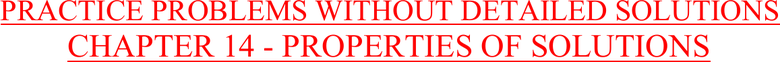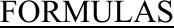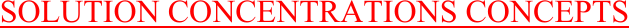Study Guides (380,000)
US (220,000)
Rutgers (3,000)
3:40 (100)
Final

01:160:161 Chapter Notes - Chapter 14: Colligative Properties, Mole Fraction, Boiling PointExam

Department
Chemistry
Course Code
01:160:161
Professor
Asbed Vassilia
Study Guide
Final

This preview shows pages 1-3. to view the full 41 pages of the document.1
PRACTICE PROBLEMS WITHOUT DETAILED SOLUTIONS
CHAPTER 14 - PROPERTIES OF SOLUTIONS
SOLUTION CONCENTRATIONS CONCEPTS (Molarity, mole fraction, etc.)
SOLUTION CONCENTRATIONS CALCULATIONS (Molarity, mole fraction, etc.)
ENERGETICS OF SOLUTIONS AND SOLUBILITY CONCEPTS
ENERGETICS OF SOLUTIONS AND SOLUBILITY CALCULATIONS
VAPOR PRESSURE OF SOLUTIONS CONCEPTS (Henry’s Law, Raoult’s Law)
VAPOR PRESSURE OF SOLUTIONS CALCULATIONS
COLLIGATIVE PROPERTIES (BOILING POINT, FREEZING POINT, OSMOTIC PRESSURE
CHANGES) CONCEPTS
COLLIGATIVE PROPERTIES (BOILING POINT, FREEZING POINT, OSMOTIC PRESSURE
CHANGES) CALCULATIONS
MISCELLANEOUS (INCLUDING COLLOIDS)
E. Tavss, PhD

Only pages 1-3 are available for preview. Some parts have been intentionally blurred.2
FORMULAS
Mass percent = grams of solute/100 g solution
Molarity = moles of solute/L of solution
Molality = moles of solute/kilogram of solvent
PPM = grams of solute/1,000,000 grams solution
Volume percent = volume of solute/100 mL of solution
Proof = 2 x Vol. %; e.g., 2 x 40 mL/100 mL solution = 80 proof
Mole fraction : XA = nA/(nA + nB) XA + XB = 1
Particle fraction : iXA = inA/(inA + inB) iXA + iXB = 1
Raoult’s law: Psoln = iXsolventPo
solvent
iXsolvent = insolvent/(insolvent + insolute)
iXA + iXB = 1
PTotal = PA + PB = iXAPo
A + iXBPo
B
van’t Hoff factor, “i”; i = moles of particles in solution/moles of solute dissolved
Boiling-point elevation: ΔT = Tf – Ti = Kbimsolute
Freezing-point depression: ΔT = Tf – Ti = -Kfimsolute
(+Kf gives absolute change in temp; -Kf gives actual Tf or Ti)
Osmotic pressure: πV = inRT
π = (n/V)iRT = iMsoluteRT
ΔHsoln = ΔHsolute-solutebondbreaking + ΔHsolvent-solventbondbreaking + ΔHsolute-solventbondforming
ΔHhydr = ΔHsolvent-solventbondbreaking + ΔHsolute-solventbondforming
ΔHsoln = ΔHsolute-solutebondbreaking + ΔHhydr
Henry’s Law: XA=kPA; XA = mole fraction of dissolved gas in solution

Only pages 1-3 are available for preview. Some parts have been intentionally blurred.3
SOLUTION CONCENTRATIONS CONCEPTS
CHEM 162-2010 EXAM I
Chapter 12 – Properties of Solutions
7. A solution can be carefully prepared to hold more solute than the maximum solubility. Such a solution
is called
A. supersaturated
B. subsaturated
C. saturated
D. isotonic
E. hypertonic
CHEM 162-2009 EXAM I
CHAPTER 12 – PROPERTIES OF SOLUTIONS
21. Which of the following statements is true regarding molality and molarity?
A. Neither molality nor molarity depend on temperature.
B. Both molality and molarity depend on temperature.
C. Molarity depends on temperature but molality does not.
D. Molality depends on temperature but molarity does not.
E. Temperature dependence of molality and molarity depends on the material.